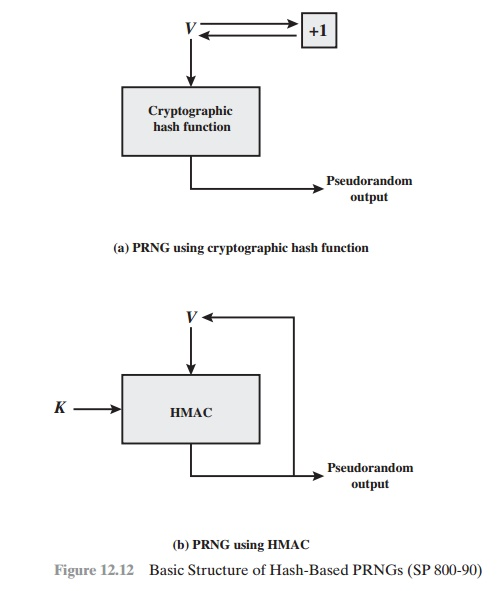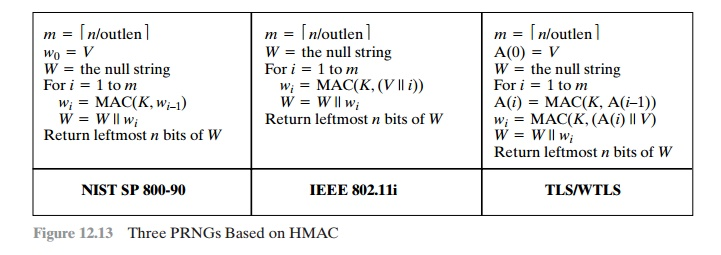Home | | Cryptography and Network Security | Pseudorandom Number Generation Using Hash Functions and MACS

# Pseudorandom Number Generation Using Hash Functions and MACS

The essential elements of any pseudorandom number generator (PRNG) are a seed value and a deterministic algorithm for generating a stream of pseudorandom bits.

PSEUDORANDOM NUMBER GENERATION USING HASH FUNCTIONS AND MACS

The essential elements of any pseudorandom number generator (PRNG) are a seed value and a deterministic algorithm for generating a stream of pseudorandom bits. If the algorithm is used as a pseudorandom function (PRF) to produce a required value, such as a session key, then the seed should only be known to the user of the PRF. If the algorithm is used to produce a stream encryption function, then the seed has the role of a secret key that must be known to the sender and the receiver.

We noted in Chapters 7 and 10 that, because an encryption algorithm pro- duces an apparently random output, it can serve as the basis of a (PRNG). Similarly, a hash function or MAC produces apparently random output and can be used to build a PRNG. Both ISO standard 18031 (Random Bit Generation) and NIST SP 800-90 (Recommendation for Random Number Generation Using Deterministic Random Bit Generators) define an approach for random number generation using a cryptographic hash function. SP 800-90 also defines a random number generator based on HMAC. We look at these two approaches in turn.

PRNG Based on Hash Function

Figure 12.12a shows the basic strategy for a hash-based PRNG specified in SP 800-90 and ISO 18031. The algorithm takes as input:

V  = seed

seedlen = bit length of V >= k + 64,  where   k is   a   desired   security level expressed in bits

n = desired number of output bitsThe algorithm uses the cryptographic hash function H with an hash value out- put of outlen bits. The basic operation of the algorithm isThe SP 800-90 specification also provides for periodically updating V to enhance security. The specification also indicates that there are no known or sus- pected weaknesses in the hash-based approach for a strong cryptographic hash algorithm, such as SHA-2.

PRNG Based on MAC Function

Although there are no known or suspected weaknesses in the use of a cryptographic hash function for a PRNG in the manner of Figure 12.12a, a higher degree of confi- dence can be achieved by using a MAC. Almost invariably, HMAC is used for constructing a MAC-based PRNG. This is because HMAC is a widely used stan- dardized MAC function and is implemented in many protocols and applications. As SP 800-90 points out, the disadvantage of this approach compared to the hash-based approach is that the execution time is twice as long, because HMAC involves two executions of the underlying hash function for each output block. The advantage of the HMAC approach is that it provides a greater degree of confidence in its security, compared to a pure hash-based approach.

For the MAC-based approach, there are two inputs: a key K and a seed V. In effect, the combination of K and V form the overall seed for the PRNG specified in SP 800-90. Figure 12.12b shows the basic structure of the PRNG mechanism, and the leftmost column of Figure 12.13 shows the logic. Note that the key remains the same for each block of output, and the data input for each block is equal to the tag output of the previous block. The SP 800-90 specification also provides for periodically updating K and V to enhance security.

It is instructive to compare the SP 800-90 recommendation with the use of HMAC for a PRNG in some applications, and this is shown in Figure 12.13. For the IEEE 802.11i wireless LAN security standard (Chapter 17), the data input consists of the seed concatenated with a counter. The counter is incremented for each block wi of output. This approach would seem to offer enhanced security compared to the SP 800-90 approach. Consider that for SP 800-90, the data input for output block wi is just the output wi - 1 of the previous execution of HMAC. Thus, an opponent who is able to observe the pseudorandom output knows both the input and output ofHMAC. Even so, with the assumption that HMAC is secure, knowledge of the input and output should not be sufficient to recover K and hence not sufficient to predict future pseudorandom bits.

The approach taken by the Transport Layer Security protocol (Chapter 16) and the Wireless Transport Layer Security Protocol (Chapter 17) involves invoking HMAC twice for each block of output wi. As with IEEE 802.11, this is done in such a way that the output does not yield direct information about the input. The double use of HMAC doubles the execution burden and would seem to be security overkill.

Study Material, Lecturing Notes, Assignment, Reference, Wiki description explanation, brief detail
Cryptography and Network Security Principles and Practice : Cryptographic Data Integrity Algorithms : Message Authentication Codes : Pseudorandom Number Generation Using Hash Functions and MACS |

Related Topics